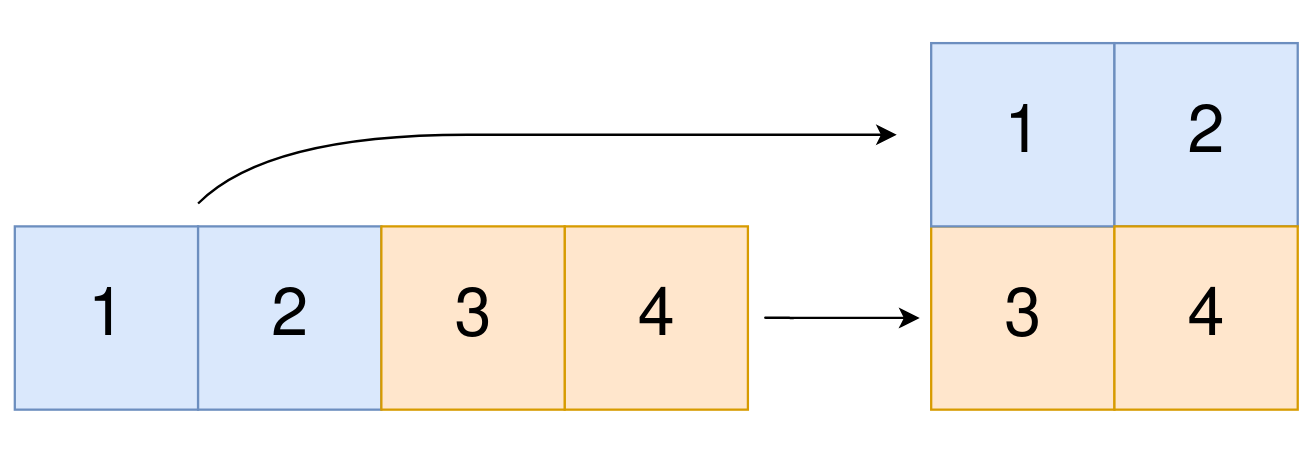2022. Convert 1D Array Into 2D Array

You are given a 0-indexed 1-dimensional (1D) integer array `original`, and two integers, `m` and `n`. You are tasked with creating a 2-dimensional (2D) array with ` m` rows and `n` columns using all the elements from `original`.

The elements from indices `0` to `n - 1` (inclusive) of `original` should form the first row of the constructed 2D array, the elements from indices `n` to `2 * n - 1` (inclusive) should form the second row of the constructed 2D array, and so on.

Return an `m x n` 2D array constructed according to the above procedure, or an empty 2D array if it is impossible.

Example 1:```Input: original = [1,2,3,4], m = 2, n = 2
Output: [[1,2],[3,4]]
Explanation:
The constructed 2D array should contain 2 rows and 2 columns.
The first group of n=2 elements in original, [1,2], becomes the first row in the constructed 2D array.
The second group of n=2 elements in original, [3,4], becomes the second row in the constructed 2D array.
```

Example 2:

```Input: original = [1,2,3], m = 1, n = 3
Output: [[1,2,3]]
Explanation:
The constructed 2D array should contain 1 row and 3 columns.
Put all three elements in original into the first row of the constructed 2D array.
```

Example 3:

```Input: original = [1,2], m = 1, n = 1
Output: []
Explanation:
There are 2 elements in original.
It is impossible to fit 2 elements in a 1x1 2D array, so return an empty 2D array.
```

Example 4:

```Input: original = , m = 1, n = 2
Output: []
Explanation:
There is 1 element in original.
It is impossible to make 1 element fill all the spots in a 1x2 2D array, so return an empty 2D array.
```

Constraints:

• `1 <= original.length <= 5 * 104`
• `1 <= original[i] <= 105`
• `1 <= m, n <= 4 * 104`

2022. Convert 1D Array Into 2D Array
``````struct Solution;

impl Solution {
fn construct2_d_array(original: Vec<i32>, m: i32, n: i32) -> Vec<Vec<i32>> {
let k = original.len();
let m = m as usize;
let n = n as usize;
if m * n == k {
let mut res = vec![];
let mut index = 0;
for _ in 0..m {
let mut row = vec![];
for _ in 0..n {
row.push(original[index]);
index += 1;
}
res.push(row);
}
res
} else {
vec![]
}
}
}

#[test]
fn test() {
let original = vec![1, 2, 3, 4];
let m = 2;
let n = 2;
let res = vec_vec_i32![[1, 2], [3, 4]];
assert_eq!(Solution::construct2_d_array(original, m, n), res);
let original = vec![1, 2, 3];
let m = 1;
let n = 3;
let res = vec_vec_i32![[1, 2, 3]];
assert_eq!(Solution::construct2_d_array(original, m, n), res);
let original = vec![1, 2];
let m = 1;
let n = 1;
let res = vec_vec_i32![];
assert_eq!(Solution::construct2_d_array(original, m, n), res);
let original = vec!;
let m = 1;
let n = 2;
let res = vec_vec_i32![];
assert_eq!(Solution::construct2_d_array(original, m, n), res);
}
``````